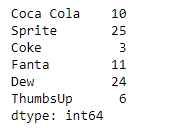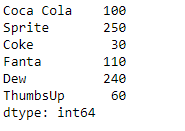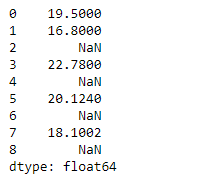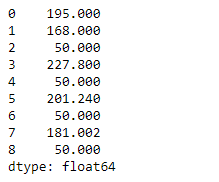Related Articles
Python | Pandas Series.multiply()
• Last Updated : 11 Feb, 2019

Pandas series is a One-dimensional ndarray with axis labels. The labels need not be unique but must be a hashable type. The object supports both integer- and label-based indexing and provides a host of methods for performing operations involving the index.

Pandas` Series.multiply()` function perform the multiplication of series and other, element-wise. The operation is equivalent to `series * other`, but with support to substitute a fill_value for missing data in one of the inputs.

Syntax: Series.multiply(other, level=None, fill_value=None, axis=0)

Parameter :
other : Series or scalar value
fill_value : Fill existing missing (NaN) value
level : Broadcast across a level,

Returns : result : Series

Example #1: Use `Series.multiply()` function to perform the multiplication of a scalar with the given series object.

 `# importing pandas as pd``import` `pandas as pd`` ` `# Creating the Series``sr ``=` `pd.Series([``10``, ``25``, ``3``, ``11``, ``24``, ``6``])`` ` `# Create the Index``index_ ``=` `[``'Coca Cola'``, ``'Sprite'``, ``'Coke'``, ``'Fanta'``, ``'Dew'``, ``'ThumbsUp'``]`` ` `# set the index``sr.index ``=` `index_`` ` `# Print the series``print``(sr)`

Output :Now we will use `Series.multiply()` function to perform the multiplication of scalar with the series.

 `# multiply the given value with series``result ``=` `sr.multiply(other ``=` `10``)`` ` `# Print the result``print``(result)`

Output :As we can see in the output, the `Series.multiply()` function has returned the result of multiplication of the given scalar with the series object.

Example #2: Use `Series.multiply()` function to perform the multiplication of a scalar with the given series object. The given series object contains some missing values.

 `# importing pandas as pd``import` `pandas as pd`` ` `# Creating the Series``sr ``=` `pd.Series([``19.5``, ``16.8``, ``None``, ``22.78``, ``None``, ``20.124``, ``None``, ``18.1002``, ``None``])`` ` `# Print the series``print``(sr)`

Output :Now we will use `Series.multiply()` function to perform the multiplication of scalar with the series.

 `# multiply the given value with series``# fill 5 at the place of all the missing values``result ``=` `sr.multiply(other ``=` `10``, fill_value ``=` `5``)`` ` `# Print the result``print``(result)`

Output :As we can see in the output, the `Series.multiply()` function has returned the result of multiplication of the given scalar with the series object.

Attention geek! Strengthen your foundations with the Python Programming Foundation Course and learn the basics.

To begin with, your interview preparations Enhance your Data Structures concepts with the Python DS Course.

My Personal Notes arrow_drop_up